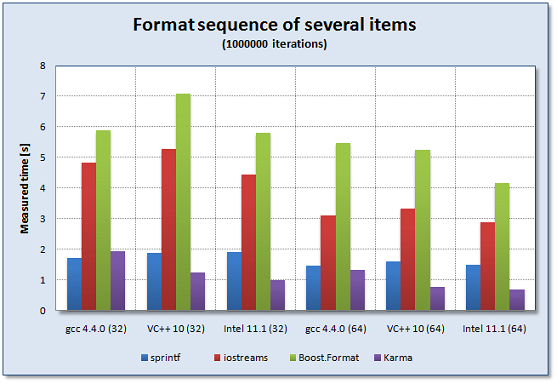#Boost C++ Libraries

...one of the most highly regarded and expertly designed C++ library projects in the world.

##### Comparing the performance of a sequence of several generators

These performance measurements are centered around formatting of a sequence of different items, including 2 `double` floating point numbers using different libraries and methods. The overall execution times for those examples are compared below. We compare using `sprintf`, C++ iostreams, Boost.Format, and Spirit.Karma.

For the full source code of the performance test please see here: format_performance.cpp. All the measurements have been done by doing `1e6` iterations for each formatting type (NUMITERATIONS is set to `1e6`).

Code used to measure the performance for sprintf:

```char buffer;
for (int i = 0; i < NUMITERATIONS; ++i) {
sprintf(buffer, "[%-14.3f%-14.3f]", 12345.12345, 12345.12345);
}
```

Code used to measure the performance for standard iostreams:

```std::stringstream strm;
for (int i = 0; i < NUMITERATIONS; ++i) {
strm.str("");
strm << '['
<< std::setiosflags(std::ios::fixed)
<< std::left
<< std::setprecision(3)
<< std::setw(14)
<< 12345.12345
<< std::setw(14)
<< 12345.12345
<< ']';
}
```

Code used to measure the performance for Boost.Format:

```std::string generated;
boost::format outformat("[%-14.3f%-14.3f]");
for (int i = 0; i < NUMITERATIONS; ++i)
generated = boost::str(outformat % 12345.12345 % 12345.12345);
```

The following code shows the common definitions used by all Spirit.Karma performance measurements as listed below:

```template <typename T>
struct double3_policy : boost::spirit::karma::real_policies<T>
{
//  we want to generate up to 3 fractional digits
static unsigned int precision(T) { return 3; }
};

typedef boost::spirit::karma::real_generator<double, double3_policy<double> >
double3_type;
double3_type const double3 = double3_type();
```

Code used to measure the performance for Spirit.Karma using a plain character buffer:

```char buffer;
for (int i = 0; i < NUMITERATIONS; ++i) {
char *p = buffer;
generate(p
, '[' << left_align(14)[double3] << left_align(14)[double3] << ']'
, 12345.12345, 12345.12345);
*p = '\0';
}
```

The following table shows the overall performance results collected while using different compilers. All times are in seconds measured for `1e6` iterations (platform: Windows7, Intel Core Duo(tm) Processor, 2.8GHz, 4GByte RAM). For a more readable comparison of the results see this figure.

Table 7. Performance comparison for a sequence of several items (all times in [s], `1e6` iterations)

Library

gcc 4.4.0 (32 bit)

VC++ 10 (32 bit)

Intel 11.1 (32 bit)

gcc 4.4.0 (64 bit)

VC++ 10 (64 bit)

Intel 11.1 (64 bit)

sprintf

1.725

1.892

1.903

1.469

1.608

1.493

iostreams

4.827

5.287

4.444

3.112

3.319

2.877

5.881

7.089

5.801

5.455

5.254

4.164

Spirit.Karma

1.942

1.242

0.999

1.334

0.758

0.686

Figure 5. Performance comparison for a sequence of several items